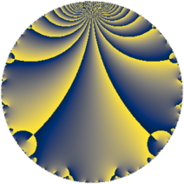# Properties

 Label 1849.4.cLevel $1849$ Weight $4$ Character orbit 1849.c Rep. character $\chi_{1849}(423,\cdot)$ Character field $\Q(\zeta_{3})$ Dimension $862$ Sturm bound $630$

# Related objects

## Defining parameters

 Level: $$N$$ $$=$$ $$1849 = 43^{2}$$ Weight: $$k$$ $$=$$ $$4$$ Character orbit: $$[\chi]$$ $$=$$ 1849.c (of order $$3$$ and degree $$2$$) Character conductor: $$\operatorname{cond}(\chi)$$ $$=$$ $$43$$ Character field: $$\Q(\zeta_{3})$$ Sturm bound: $$630$$

## Dimensions

The following table gives the dimensions of various subspaces of $$M_{4}(1849, [\chi])$$.

Total New Old
Modular forms 990 942 48
Cusp forms 902 862 40
Eisenstein series 88 80 8

## Trace form

 $$862q + 2q^{2} + 5q^{3} + 3282q^{4} + 19q^{5} - 15q^{6} + 51q^{7} + 72q^{8} - 3474q^{9} + O(q^{10})$$ $$862q + 2q^{2} + 5q^{3} + 3282q^{4} + 19q^{5} - 15q^{6} + 51q^{7} + 72q^{8} - 3474q^{9} - 27q^{10} - 74q^{11} + 72q^{12} + 19q^{13} - 96q^{14} - 65q^{15} + 11962q^{16} + 74q^{17} - 247q^{18} - 78q^{19} + 495q^{20} + 18q^{21} - 380q^{22} + 35q^{23} - 202q^{24} - 8774q^{25} + 21q^{26} + 194q^{27} + 794q^{28} + 53q^{29} - 627q^{30} - 303q^{31} + 798q^{32} + 424q^{33} + 231q^{34} - 710q^{35} - 11004q^{36} + 129q^{37} + 854q^{38} - 1382q^{39} - 1345q^{40} - 622q^{41} - 62q^{42} - 1158q^{44} - 1888q^{45} + 40q^{46} + 420q^{47} + 2401q^{48} - 15320q^{49} - 424q^{50} - 1590q^{51} + 628q^{52} - 889q^{53} - 1848q^{54} + 1242q^{55} + 1581q^{56} + 765q^{57} - 1328q^{58} + 3006q^{59} + 1565q^{60} - 437q^{61} - 1509q^{62} + 2222q^{63} + 39108q^{64} + 2126q^{65} - 1245q^{66} + 484q^{67} + 924q^{68} + 3503q^{69} + 170q^{70} + 1545q^{71} - 3834q^{72} - 1292q^{73} + 2134q^{74} - 164q^{75} + 252q^{76} - 1448q^{77} - 5644q^{78} + 1145q^{79} + 3157q^{80} - 23087q^{81} - 6608q^{82} - 749q^{83} - 6268q^{84} - 1946q^{85} - 4562q^{87} + 5372q^{88} + 2196q^{89} - 668q^{90} + 3513q^{91} - 3045q^{92} + 983q^{93} - 9878q^{94} + 739q^{95} - 4562q^{96} - 110q^{97} + 213q^{98} + 3631q^{99} + O(q^{100})$$

## Decomposition of $$S_{4}^{\mathrm{new}}(1849, [\chi])$$ into newform subspaces

The newforms in this space have not yet been added to the LMFDB.

## Decomposition of $$S_{4}^{\mathrm{old}}(1849, [\chi])$$ into lower level spaces

$$S_{4}^{\mathrm{old}}(1849, [\chi]) \cong$$ $$S_{4}^{\mathrm{new}}(43, [\chi])$$$$^{\oplus 2}$$